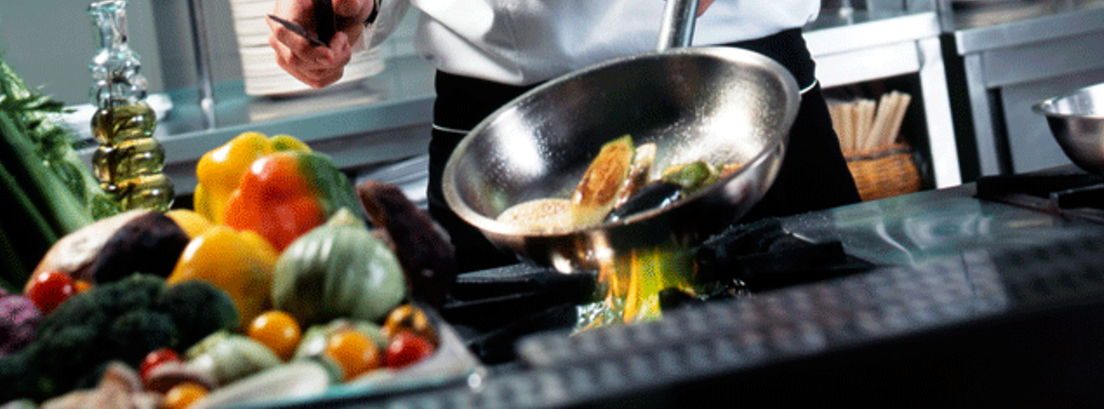# Public Voting Update of Cooking Recipe Contest 2020Dear Contestants,

The Public Voting for Cooking Recipe Contest 2020 is over now. The Public voting for the contest started on the 3rd of July till 22nd July and the response was awesome. The public voting closed at 11 PM on 22nd July.

Final vote-counting was completed by 11.01 PM. The recipes are now forwarded to the jury for judgment on other parameters. The results will be released on 2nd August 2020 ( Sunday) at 4 PM. As promised 10% of the total vote counting will be added with judges score to declare the winners. The Following score  for public votes recorded by us:

Shivee: FB(46) + Insta(4) =50 Votes =Final Voting score 5 (10% of total votes)

Laxmi Rana (Dish 1): Insta(7)+ FB(145)=152 Votes=Final Voting score 15.2 (10% of total votes)

Laxmi Rana (Dish 2): Insta(9)+ FB(56)=65 Votes=Final Voting score 6.5 (10% of total votes)

Jui Mandal: FB(27) + Insta(9)= 36 Votes=Final Voting score 3.6 (10% of total votes)

Gunjan: FB(60) + Insta(7)= 67 Votes=Final Voting score 6.7 (10% of total votes)

Moumita Paul(Dish 1)=FB(14) + Insta(8)= 20 Votes=Final Voting score 2 (10% of total votes)

Moumita Paul(Dish 2)=FB(14) + Insta(6)= 20 Votes=Final Voting score 2 (10% of total votes)

Moumita Paul(Dish 3)=FB(17) + Insta(7)= 24 Votes=Final Voting score 2.4 (10% of total votes)

Sandhya Trivedi: FB(59) + Insta(40)= 99 Votes=Final Voting score 9.9 (10% of total votes)

Arnab (Dish 1): FB(17) + Insta(4)= 21 Votes=Final Voting score 2.1 (10% of total votes)

Arnab (Dish 2): FB(17) + Insta(4)= 21 Votes=Final Voting score 2.1 (10% of total votes)

Arnab (Dish 3): FB(22) + Insta(12)= 34Votes=Final Voting score 3.4 (10% of total votes)

Bidisha Mukherjee (Dish 1): FB(12) + Insta(8)= 20Votes=Final Voting score 2 (10% of total votes)

Bidisha Mukherjee (Dish 2): FB() + Insta(7)= 9 Votes=Final Voting score 0.9 (10% of total votes)

Bidisha Mukherjee (Dish 3): FB(11) + Insta(10)= 21Votes=Final Voting score 2.1 (10% of total votes)

Ruchi Trivedi: FB(78) + Insta(9)= 87 Votes=Final Voting score 8.7 (10% of total votes)

Smita Jha: FB(120)+Insta(5)= 125 Votes=Final Voting score 12.5 (10% of total votes)

Neha Dutta (Dish 1): FB(204) + Insta(48)= 252 Votes=Final Voting score 25.2 (10% of total votes)

Neha Dutta (Dish 2): FB(192) + Insta(43)= 235 Votes=Final Voting score 23.5 (10% of total votes)

Neha Dutta (Dish 3): FB(195) + Insta(48)= 243 Votes=Final Voting score 24.3 (10% of total votes)

For any discrepancy noticed in the voting score please comment below and we will check. Only voting will not decide the winner as the Judge’s decision is major. Judges Score+Voting score= Will decide Winners.

All The Best…

Team Indiacafe24